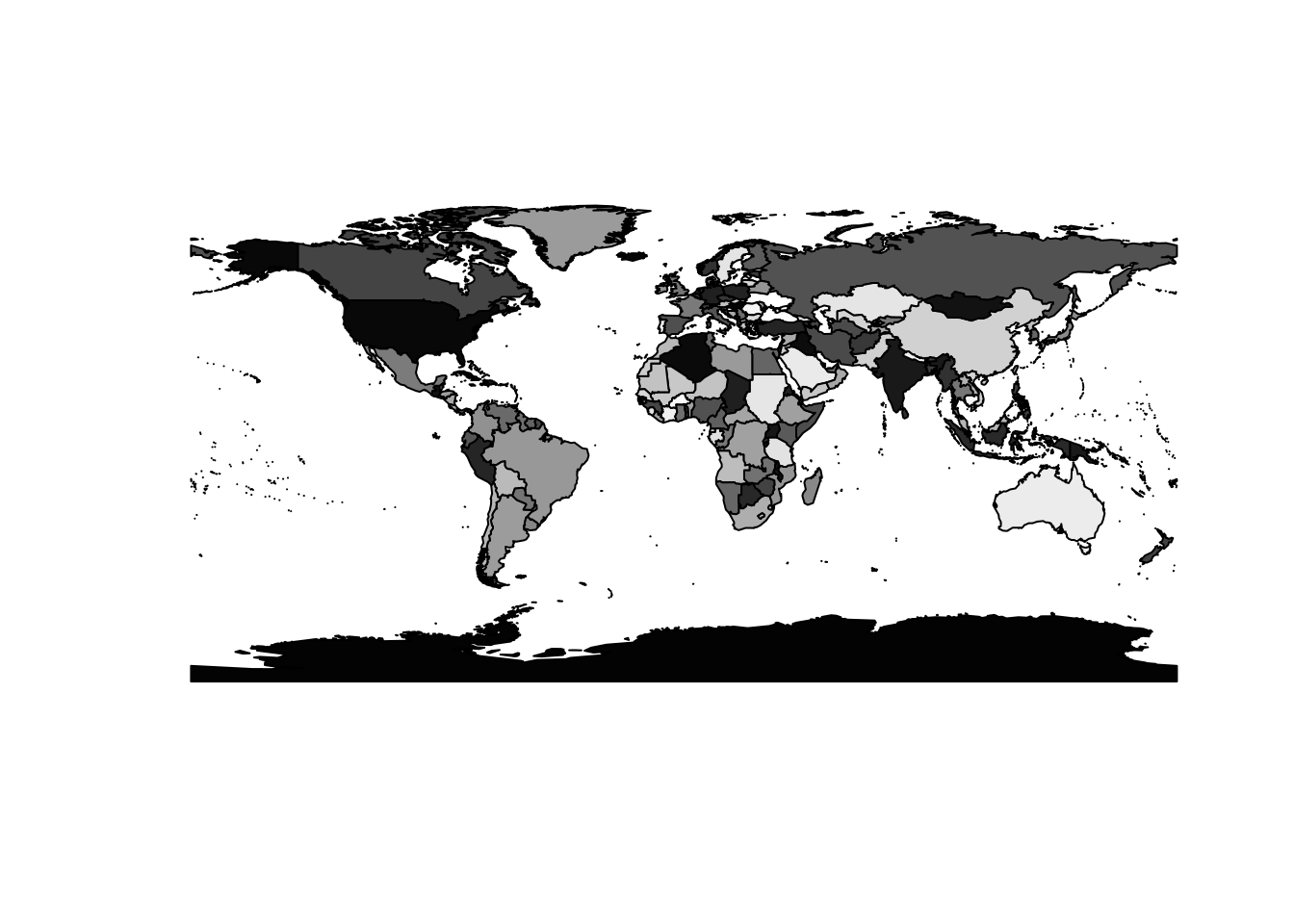# GIS for 3D in R

news
code
Author

Michael D. Sumner

Published

2015-12-28

GIS data structures are not well suited for generalization, and visualizations and models in 3D require pretty forceful and ad hoc approaches.

Here I describe a simple example, showing several ways of visualizing a simple polygon data set. I use the programming environment R for the data manipulation and the creation of this document via several extensions (packages) to base R.

## Polygon “layer”

The R package maptools contains an in-built data set called wrld_simpl, which is a basic (and out of date) set of polygons describing the land masses of the world by country. This code loads the data set and plots it with a basic grey-scale scheme for individual countries.

library(maptools)
data(wrld_simpl)
print(wrld_simpl)
class       : SpatialPolygonsDataFrame
features    : 246
extent      : -180, 180, -90, 83.57027  (xmin, xmax, ymin, ymax)
variables   : 11
# A tibble: 246 × 11
FIPS  ISO2  ISO3     UN NAME      AREA POP2005 REGION SUBREGION     LON   LAT
<fct> <fct> <fct> <int> <fct>    <int>   <dbl>  <int>     <int>   <dbl> <dbl>
1 AC    AG    ATG      28 Antigu…     44  8.30e4     19        29  -61.8   17.1
2 AG    DZ    DZA      12 Algeria 238174  3.29e7      2        15    2.63  28.2
3 AJ    AZ    AZE      31 Azerba…   8260  8.35e6    142       145   47.4   40.4
4 AL    AL    ALB       8 Albania   2740  3.15e6    150        39   20.1   41.1
5 AM    AM    ARM      51 Armenia   2820  3.02e6    142       145   44.6   40.5
6 AO    AO    AGO      24 Angola  124670  1.61e7      2        17   17.5  -12.3
7 AQ    AS    ASM      16 Americ…     20  6.41e4      9        61 -171.   -14.3
8 AR    AR    ARG      32 Argent… 273669  3.87e7     19         5  -65.2  -35.4
9 AS    AU    AUS      36 Austra… 768230  2.03e7      9        53  136.   -25.0
10 BA    BH    BHR      48 Bahrain     71  7.25e5    142       145   50.6   26.0
# … with 236 more rows
plot(wrld_simpl, col = grey(sample(seq(0, 1, length = nrow(wrld_simpl)))))We also include a print statement to get a description of the data set, this is a SpatialPolygonsDataFrame which is basically a table of attributes with one row for each country, linked to a recursive data structure holding sets of arrays of coordinates for each individual piece of these complex polygons.

These structures are quite complicated, involving nested lists of matrices with X-Y coordinates. I can use class coercion from polygons, to lines, then to points as the most straightforward way of obtaining every XY coordinate by dropping the recursive hierarchy structure to get at every single vertex in one matrix.

allcoords <- coordinates(as(as(wrld_simpl, "SpatialLines"), "SpatialPoints"))
dim(allcoords)
 26264     2
head(allcoords)  ## print top few rows
     coords.x1 coords.x2
[1,] -61.68667  17.02444
[2,] -61.88722  17.10527
[3,] -61.79445  17.16333
[4,] -61.68667  17.02444
[5,] -61.72917  17.60861
[6,] -61.85306  17.58305

(There are other methods to obtain all coordinates while retaining information about the country objects and their component “pieces”, but I’m ignoring that for now.)

We need to put these “X/Y” coordinates in 3D so I simply add another column filled with zeroes.

allcoords <- cbind(allcoords, 0)
head(allcoords)
     coords.x1 coords.x2
[1,] -61.68667  17.02444 0
[2,] -61.88722  17.10527 0
[3,] -61.79445  17.16333 0
[4,] -61.68667  17.02444 0
[5,] -61.72917  17.60861 0
[6,] -61.85306  17.58305 0

(Note for non-R users: in R expressions that don’t include assignment to an object with <- are generally just a side-effect, here the side effect of the head(allcoords) here is to print the top few rows of allcoords, just for illustration, there’s no other consequence of this code).

## OpenGL in R

In R we have access to 3D visualizations in OpenGL via the rgl package, but the model for data representation is very different so I first plot the vertices of the wrld_simpl layer as points only.

library(rgl)
plot3d(allcoords, xlab = "", ylab = "") ## smart enough to treat 3-columns as X,Y,Z
rglwidget()

Plotting in the plane is one thing, but more striking is to convert the vertices from planar longitude-latitude to Cartesizan XYZ. Define an R function to take “longitude-latitude-height” and return spherical coordinates (we can leave WGS84 for another day).

llh2xyz <-
function (lonlatheight, rad = 6378137, exag = 1)
{
cosLat = cos(lonlatheight[, 2] * pi/180)
sinLat = sin(lonlatheight[, 2] * pi/180)
cosLon = cos(lonlatheight[, 1] * pi/180)
sinLon = sin(lonlatheight[, 1] * pi/180)
rad <- (exag * lonlatheight[, 3] + rad)
x = rad * cosLat * cosLon
y = rad * cosLat * sinLon
z = rad * sinLat
cbind(x, y, z)
}

## deploy our custom function on the longitude-latitude values
xyzcoords <- llh2xyz(allcoords)

Now we can visualize these XYZ coordinates in a more natural setting, and even add a blue sphere for visual effect.

plot3d(xyzcoords, xlab = "", ylab = "")
spheres3d(0, 0, 0, radius = 6370000, col = "lightblue")
rglwidget()